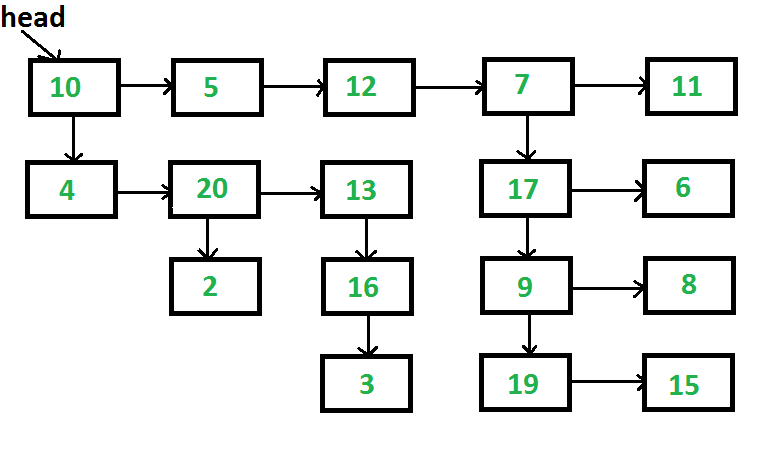GeeksforGeeks App
Open AppBrowser
Continue

# C Program For Flattening A Multilevel Linked List

Given a linked list where in addition to the next pointer, each node has a child pointer, which may or may not point to a separate list. These child lists may have one or more children of their own, and so on, to produce a multilevel data structure, as shown in below figure.You are given the head of the first level of the list. Flatten the list so that all the nodes appear in a single-level linked list. You need to flatten the list in way that all nodes at first level should come first, then nodes of second level, and so on.
Each node is a C struct with the following definition.

## C

 `struct` `List``{``    ``int` `data;``    ``struct` `List *next;``    ``struct` `List *child;``};`The above list should be converted to 10->5->12->7->11->4->20->13->17->6->2->16->9->8->3->19->15

The problem clearly says that we need to flatten level by level. The idea of a solution is, we start from the first level, process all nodes one by one, if a node has a child, then we append the child at the end of the list, otherwise, we don’t do anything. After the first level is processed, all next-level nodes will be appended after the first level. The same process is followed for the appended nodes.

```1) Take "cur" pointer, which will point to head of the first level of the list
2) Take "tail" pointer, which will point to end of the first level of the list
3) Repeat the below procedure while "curr" is not NULL.
I) if current node has a child then
a) append this new child list to the "tail"
tail->next = cur->child
b) find the last node of new child list and update "tail"
tmp = cur->child;
while (tmp->next != NULL)
tmp = tmp->next;
tail = tmp;
II) move to the next node. i.e. cur = cur->next```

Following is the implementation of the above algorithm.

## C

 `// Program to flatten list with next``// and child pointers``#include ``#include ` `// Macro to find number of elements``// in array``#define SIZE(arr) (sizeof(arr)/``                   ``sizeof``(arr))` `// A linked list node has data,``// next pointer and child pointer``struct` `Node``{``    ``int` `data;``    ``struct` `Node *next;``    ``struct` `Node *child;``};` `// A utility function to create a linked list``// with n nodes. The data of nodes is taken``// from arr[].  All child pointers are set as NULL``struct` `Node *createList(``int` `*arr, ``int` `n)``{``    ``struct` `Node *head = NULL;``    ``struct` `Node *p;` `    ``int` `i;``    ``for` `(i = 0; i < n; ++i)``    ``{``        ``if` `(head == NULL)``            ``head = p = (``struct` `Node *)``malloc``(``sizeof``(*p));``        ``else``        ``{``            ``p->next = (``struct` `Node *)``malloc``(``sizeof``(*p));``            ``p = p->next;``        ``}``        ``p->data = arr[i];``        ``p->next = p->child = NULL;``    ``}``    ``return` `head;``}` `// A utility function to print all nodes``// of a linked list``void` `printList(``struct` `Node *head)``{``    ``while` `(head != NULL)``    ``{``        ``printf``(``"%d "``, head->data);``        ``head = head->next;``    ``}``    ``printf``(``""``);``}` `// This function creates the input list. ``// The created list is same as shown in``// the above figure``struct` `Node *createList(``void``)``{``    ``int` `arr1[] = {10, 5, 12, 7, 11};``    ``int` `arr2[] = {4, 20, 13};``    ``int` `arr3[] = {17, 6};``    ``int` `arr4[] = {9, 8};``    ``int` `arr5[] = {19, 15};``    ``int` `arr6[] = {2};``    ``int` `arr7[] = {16};``    ``int` `arr8[] = {3};` `    ``// Create 8 linked lists``    ``struct` `Node *head1 = createList(arr1,``                                    ``SIZE(arr1));``    ``struct` `Node *head2 = createList(arr2,``                                    ``SIZE(arr2));``    ``struct` `Node *head3 = createList(arr3,``                                    ``SIZE(arr3));``    ``struct` `Node *head4 = createList(arr4,``                                    ``SIZE(arr4));``    ``struct` `Node *head5 = createList(arr5,``                                    ``SIZE(arr5));``    ``struct` `Node *head6 = createList(arr6,``                                    ``SIZE(arr6));``    ``struct` `Node *head7 = createList(arr7,``                                    ``SIZE(arr7));``    ``struct` `Node *head8 = createList(arr8,``                                    ``SIZE(arr8));` `    ``// Modify child pointers to create the``    ``// list shown above``    ``head1->child = head2;``    ``head1->next->next->next->child = head3;``    ``head3->child = head4;``    ``head4->child = head5;``    ``head2->next->child = head6;``    ``head2->next->next->child = head7;``    ``head7->child = head8;` `    ``/* Return head pointer of first linked list. ``       ``Note that all nodes are reachable from``       ``head1 */``    ``return` `head1;``}` `/* The main function that flattens a``   ``multilevel linked list */``void` `flattenList(``struct` `Node *head)``{``    ``// Base case``    ``if` `(head == NULL)``       ``return``;` `    ``struct` `Node *tmp;` `    ``/* Find tail node of first level``       ``linked list */``    ``struct` `Node *tail = head;``    ``while` `(tail->next != NULL)``        ``tail = tail->next;` `    ``// One by one traverse through all nodes``    ``// of first level linked list till we``    ``// reach the tail node``    ``struct` `Node *cur = head;``    ``while` `(cur != tail)``    ``{``        ``// If current node has a child``        ``if` `(cur->child)``        ``{``            ``// then append the child at the``            ``// end of current list``            ``tail->next = cur->child;` `            ``// and update the tail to new     ``            ``// last node``            ``tmp = cur->child;``            ``while` `(tmp->next)``                ``tmp = tmp->next;``            ``tail = tmp;``        ``}` `        ``// Change current node``        ``cur = cur->next;``    ``}``}` `// Driver code``int` `main(``void``)``{``    ``struct` `Node *head = NULL;``    ``head = createList();``    ``flattenList(head);``    ``printList(head);``    ``return` `0;``}`

Output:

`10 5 12 7 11 4 20 13 17 6 2 16 9 8 3 19 15`

Time Complexity: Since every node is visited at most twice, the time complexity is O(n) where n is the number of nodes in given linked list.

Space complexity:  O(n),where n is the number of nodes in the linked list. This is because the code dynamically allocates memory for each node in the linked list and does not have any additional data structures that would cause an increase in space usage.

Please refer complete article on Flatten a multilevel linked list for more details!

My Personal Notes arrow_drop_up